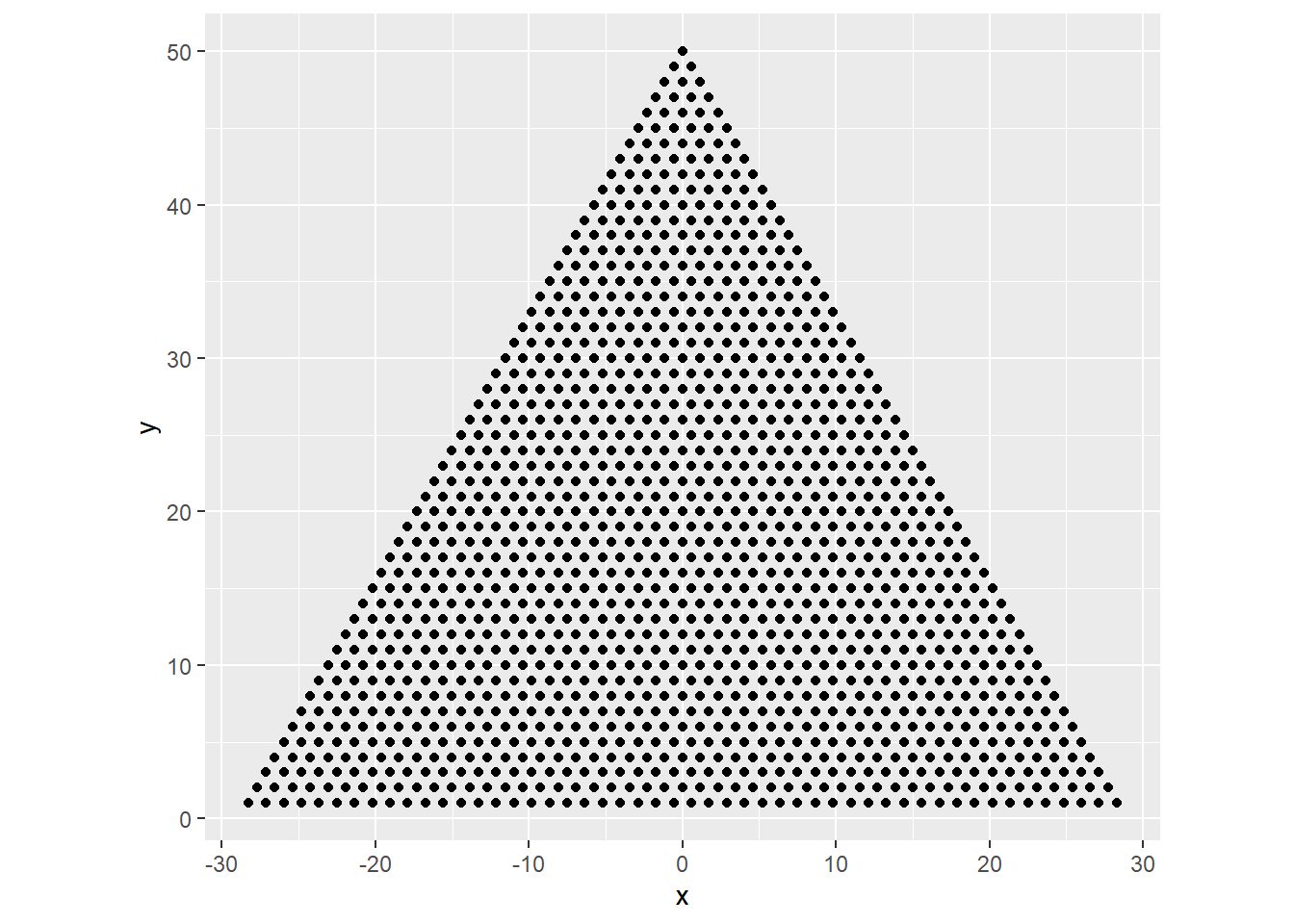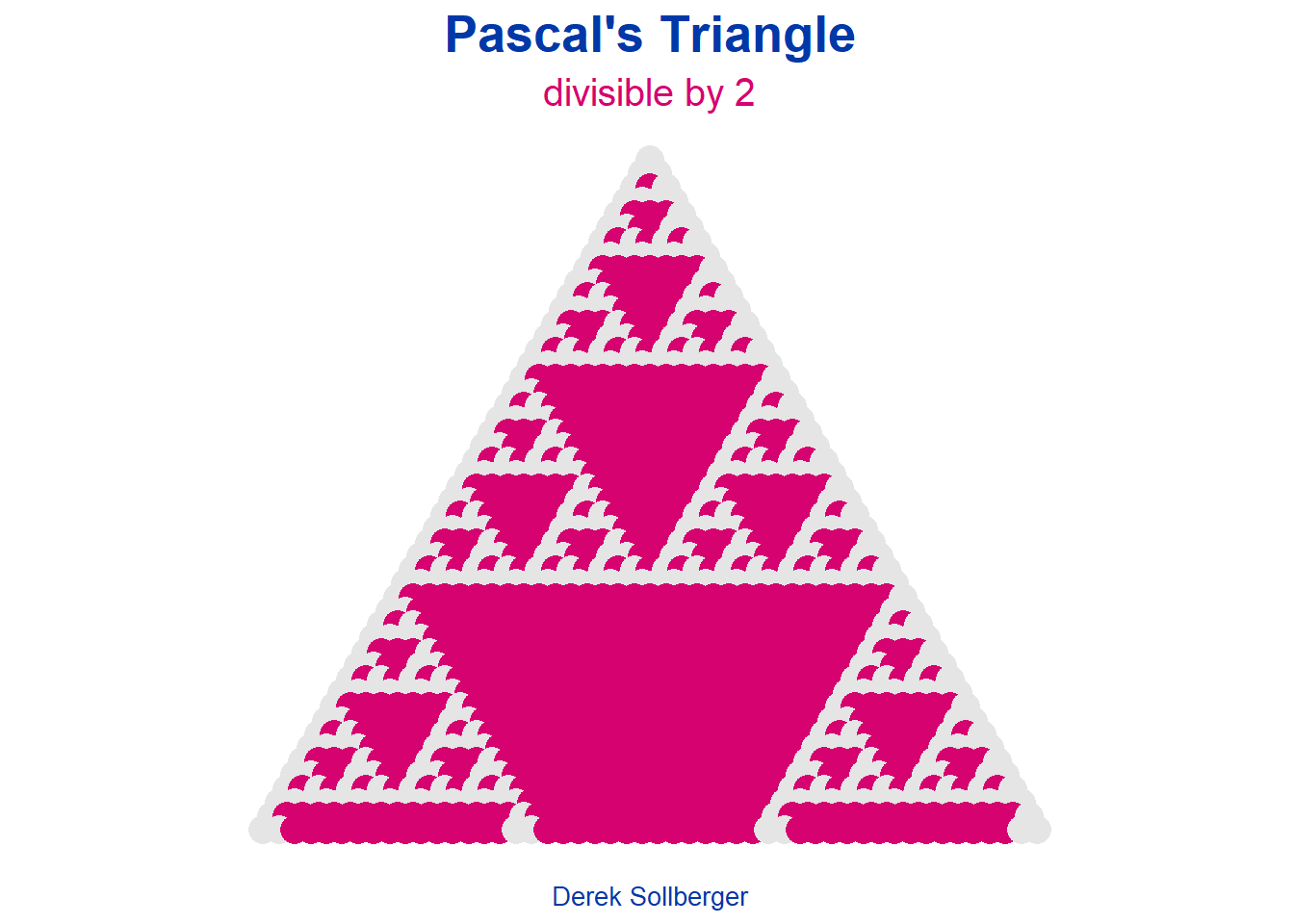# Pascal's Triangle

| July 8, 2022

Once again, I find that I have been nerd sniped (inspired by Mathigon). Today, I want to plot (and make an animation) for Pascal’s Triangle mod n. What I am thinking is

• store Pascal Triangle numbers along with row and column numbers in a data frame
• then assign x and y values
• rescale the x and y values to make an equilateral triangle
# load package(s)
library("tidyverse")
# allocate data space
N <- 50 #number of rows of wanted Pascal's Triangle
# EDIT: my computer or software did not seem to handle larger numbers well
row_total <- N*(N+1) / 2 #sum of 1 + 2 + ... + N

row_num <- rep(0, row_total)
col_num <- rep(0, row_total)
PT_val  <- rep(0, row_total)
x       <- rep(0, row_total)
y       <- rep(0, row_total)

df <- data.frame(row_num, col_num, PT_val, x, y)

Let us pretend we are back in our high school programming class and are asked to code up Pascal’s Triangle. [What’s missing here is all of my simple errors.]

# compute row and column numbers for data frame
m <- 1 #row counter in data frame
for(i in 1:N){
for(j in 1:i){
df$row_num[m] <- i df$col_num[m] <- j
m <- m + 1
}
}

# compute Pascal Triangle values
df$PT_val[df$row_num == 1 & df$col_num == 1] <- 1 df$PT_val[df$row_num == 2 & df$col_num == 1] <- 1
df$PT_val[df$row_num == 2 & df$col_num == 2] <- 1 for(i in 3:N){ for(j in 1:i){ if(j == 1){ df$PT_val[df$row_num == i & df$col_num == j] <- 1
} else if(j == i) {
df$PT_val[df$row_num == i & df$col_num == j] <- 1 } else { df$PT_val[df$row_num == i & df$col_num == j] <-
df$PT_val[df$row_num == (i-1) & df$col_num == (j-1)] + df$PT_val[df$row_num == (i-1) & df$col_num == j]
}
}
}

The $$y$$ values are easy. I simply need to reverse the order of the row numbers.

df$y <- N - df$row_num + 1

There is probably a clever way of creating $$x$$ values (toward a visual plot), but I am thinking of a brute force process

• row $$m$$ has $$m$$ non-zero Pascal’s Triangle numbers in it
• we can make a simple array 1, 2, …, $$m$$ [actually, going to count by twos]
• then shift the array so that it is centered at $$x = 0$$
for(i in 1:N){
these_x_vals <- seq(1, 2*i, 2) - i
for(j in 1:i){
df$x[df$row_num == i & df$col_num == j] <- these_x_vals[j] } } I want my graphs to make an equilateral triangle, so I need to rescale the $$x$$ values by a factor of $\frac{1}{2} \div \frac{\sqrt{3}}{2} = \frac{1}{2} * \frac{2}{\sqrt{3}} = \frac{1}{\sqrt{3}}$ df$x <- df\$x / sqrt(3)

Let’s make a graph!

df |>
ggplot() +
geom_point(aes(x = x, y = y)) +
coord_equal() #ensuring equal scales for x and yNow for the nerdy part! We can compute the Pascal Triangle numbers modulo $$n$$ for some natural number $$n$$, and the truly divisible numbers create fractal patterns.

n <- 2
df |>
ggplot() +
geom_point(aes(x = x, y = y,
color = (PT_val %% n) == 0),
size = 5) +
coord_equal() +
labs(title = "Pascal's Triangle",
subtitle = paste("divisible by", n),
caption = "Derek Sollberger") +
scale_color_manual(values = c("gray90", "#D60270")) +
theme(axis.line = element_blank(),
axis.text = element_blank(),
axis.ticks = element_blank(),
axis.title = element_blank(),
legend.position = "none",
panel.background = element_blank(),
plot.title = element_text(face = "bold", size = 20,
color = "#0038A8", hjust = 0.5),
plot.subtitle = element_text(size = 15,
color = "#D60270", hjust = 0.5),
plot.caption = element_text(size = 10,
color = "#0038A8", hjust = 0.5))To make an animation, I tend to elect to make several images, and then use a 3rd-party app to combine the images.

for(n in 2:20){
this_plot <- df |>
ggplot() +
geom_point(aes(x = x, y = y,
color = (PT_val %% n) == 0),
size = 1) +
coord_equal() +
labs(title = "Pascal's Triangle",
subtitle = paste("divisible by", n),
caption = "Derek Sollberger") +
scale_color_manual(values = c("gray75", "#D60270")) +
theme(axis.line = element_blank(),
axis.text = element_blank(),
axis.ticks = element_blank(),
axis.title = element_blank(),
legend.position = "none",
panel.background = element_blank(),
plot.title = element_text(face = "bold", size = 15,
color = "#0038A8", hjust = 0.5),
plot.subtitle = element_text(size = 15,
color = "#D60270", hjust = 0.5),
plot.caption = element_text(size = 10,
color = "#0038A8", hjust = 0.5))

# save this plot
ggsave(paste0("pascal_plot_", n, ".png"), plot = this_plot,
device = "png", height = 800, width = 1200,
dpi = 300, units = "px")
}